Next: NUMERICAL APPLICATION Up: NORMAL MODES OF VORTICES Previous: Matrix Equations

# NORMALIZATION, SPIN EXPECTATIONS AND FLUCTUATIONS

The complete eigenspectrum will contain all the information needed to determine the expectation values and fluctuations of the individual spins in the system, either for a single spinwave mode, or, for the system in thermal equilibrium. To determine expectation values of the spin components or their squares, we need to know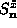and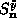in terms of the normal modes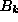and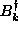. This means we need to invert the defining relations (2.12). First of all, the overall normalization ofandmust be chosen so that their commutator is unity,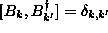. From the definitions, we must require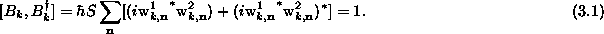where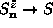was used. We assume in what follows that the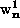andcoefficients are now rescaled to give the unit normalization and unit commutator ofwithin Eq. (3.1). Then, the following inverse expressions are assumed,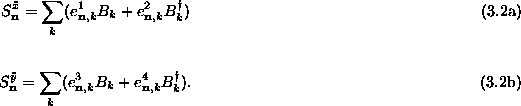To determine the new coefficients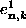and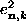in terms of the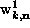and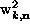coefficients, one can form the commutator ofor[Eqs. (2.12)] withand with, giving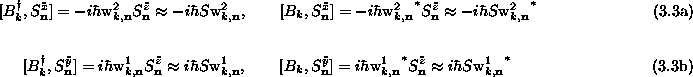On the other hand, forming the commutator ofandwithand[Eq. (3.2)] leads to equivalent results,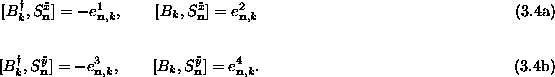Thus there is the conversion between the coefficients;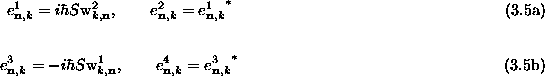As an application of these results, we can determine the local magnetization for a site by finding an expectation value of the original lab frame spin components, to quadratic order in the creation and annihilation operators. In order to do this, we first need expectation values of the spin components in the tilde coordinate system. From their definitions,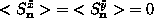, because these are linear inand. However, thecomponent will be reduced slightly below S due to spin fluctuations of the modes. In order to preserve the overall spin length and the commutation relations ofandwith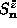, it is necessary to use the following expression for(as in the standard Holstein-Primakoff  transformation):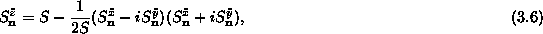where the latter terms are the spin lowering and raising operators. Using Eq. (3.2) and Eq. (3.5), the expectation value of this expression is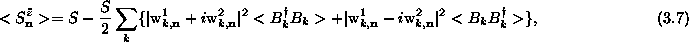where terms linear in theandoperators and terms like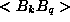andare zero in the unperturbed (single-vortex) state and in single-quantum states and therefore do not appear here. The expectation values of theandoperators will be determined by the type of state, whether it be a state with one mode excited or a thermodynamic ensemble of states (equilibrium state). For example, if the state that we are perturbing from (single vortex) is denoted |0>, then single-quantum excited states are denoted, |k>,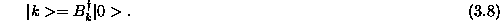The fundamental expectation values are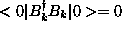, and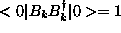. On the other hand, if the interest is in a thermal ensemble, then the expectation value required will be the Bose-Einstein occupation,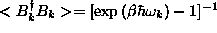, where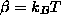is the inverse temperature. It is clear that the expectation values of the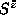components will be less than S as a result of fluctuations, while the expectation values of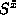and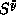will be zero. As a result, it is straightforward to use Eq. (3.7) in the coordinate transformation Eq. (2.6) to obtain the expectation values in the original lab coordinates, i.e., the spin is just reduced in effective length,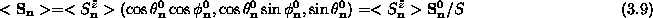We also want to know the spin fluctuations associated with some state. The spin fluctuations will be defined in terms of squares of Cartesian spin components, relative to the vortex state. For instance, the in-plane and out-of-plane spin fluctuations are described by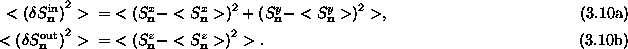Using the definitions of the tilde coordinates, Eq. (2.6), these are equivalent to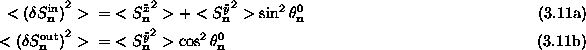Making use of the expansion of spin components in the operatorsand, Eq. (3.2), together with Eq. (3.5), one can write the fluctuations in the tilde coordinates,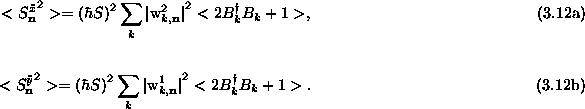Finally, the resulting in-plane and out-of-plane fluctuations are: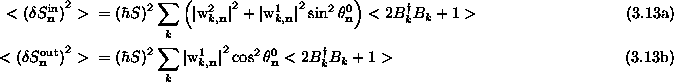Next: NUMERICAL APPLICATION Up: NORMAL MODES OF VORTICES Previous: Matrix Equations

Gary M Wysin
Mon Sep 11 12:02:10 CDT 1995